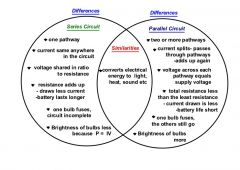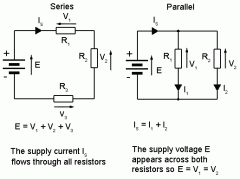• Shuffle
Toggle On
Toggle Off
• Alphabetize
Toggle On
Toggle Off
• Front First
Toggle On
Toggle Off
• Both Sides
Toggle On
Toggle Off
Toggle On
Toggle Off
Front

## Card Range To Study

throughPlay buttonPlay buttonProgress

1/16

Click to flip

Use LEFT and RIGHT arrow keys to navigate between flashcards;

Use UP and DOWN arrow keys to flip the card;

H to show hint;

 Ohm's Law V=IRV= electrical potential difference (voltage in volts)I= current in ampsR= resistance in ohms V I R Resistance R=p.(L/A)R=resistance in ohmsp= resistivity value (ohms.m)L= length (m)A= cross-sectional area (m2) Voltage-Current-Resistance Relationship 2 variables for Current:Inversely proportional to resistanceDirectly proportional to electric potential difference (delta V) Conductor Insulator Conductor - allows electric charge to flow through it Insulator - material in which e-'s are tightly bound E = elemental electric charge E= 1.6*10^-19 coulombs Coulomb Ampere Volt Coulomb = 1ampere*1 second Ampere = 1 coulomb/second = 6.25*10^18 electrons/second Volt = 1 Joule/1 Coulomb CurrentDefinitionEquation Rate at which charge flows past a point on the circuit I=Q/tI= current (ampere,A)Q= 1.6*10^-19 C (charge on 1 electron)t= time (seconds) VoltageSeries and parallelUnits Series: Vtotal = V1 + V2 + V3.... Parallel: Vtotal = V1 = V2 = V3.... Units: Volts Coulomb's Law of Electrostatic force FE = K(q1q2/r^2)FE = electrostatic forceK= 9*10^9 N.m^2/C^2 (coulomb's constant)q1 & q2 = chargesr = distance between two charges When q1 &q2 are both POSITIVE or both NEGATIVE so is FE. When they are neutral, FE=0.Halving distance quadruples the force. Doubling it quarters the force. CapacitorsCapacitanceEquation and units Capacitors - store chargeCapacitance - measure of stored charge C=Q/V Q= charge stored on one side of a capacitorV= voltage across capacitorC= capacitance (Farad)Farad= coulombs/volts Capacitors in series and parallel Series: 1/Ctotal = 1/C1 + 1/C2 +1/C3... Parallel: Ctotal = C1+ C2 +C3..... Resistance in series and parallel Series: Rtotal = R1+ R2 + R3.... Parallel: 1/Rtotal = 1/R1 + 1/R2 +1/R3.... In parallel total resistance goes downUNITS = ohms Current in series and parallel Series: I1 = I2 = I3..... Parallel: Itotal= I1 + I2 + I3..... UNITS: ampere (A) Power (electricityDefinitionUnitsEquation Definition: rate at which energy is supplied or converted by the appliance or circuitP= delta E/tP= delta V (Q/t) = delta V*I = delta V^2/Rdelta V= voltage in volts I= current in ampsR= resistance in Ohms Q = charge in coulombst= time in seconds P= power in wattsUNITS: watts (W)Uniform magnetic field Thumb=V, fingers=B, palm=FPositive= use right handNegative=use left handNo force when V is not perpendicular to B (velocity is in the same plane) F=qVBF=qVBsinthetaq= magnitude of the charge (coulombs)v= velocity of the charge (m/s)B= magnetic field vector (Teslas) Tesla= 1N/Coulomb.m/sec